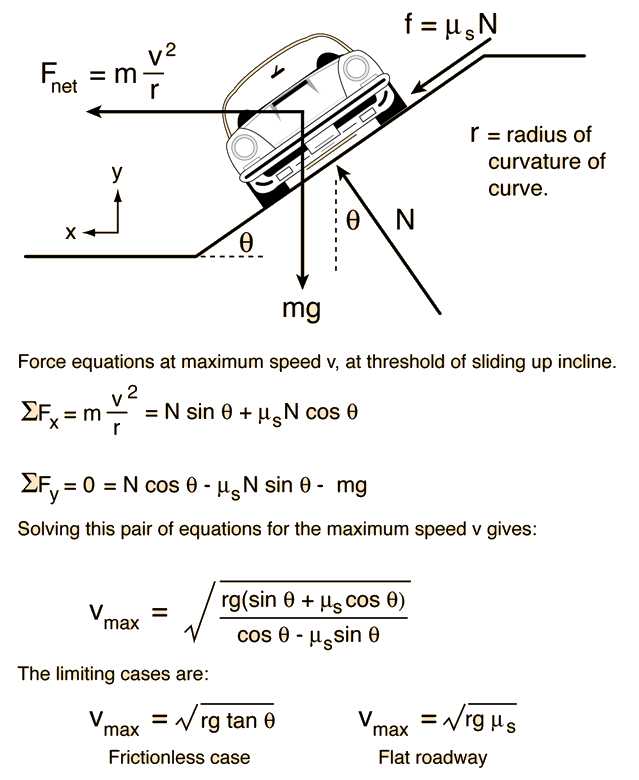# Maximum Speed on Banked RoadwayFor a highway curve of radius r = m = ft

where the angle of bank is θ = °

and the coefficient of static friction is μs = ,

the maximum speed for the banked road with this coefficient of friction is
vmax = m/s = mi/hr = km/hr.

For comparison, the maximum speed with zero friction would be
vmax = m/s = mi/hr = km/hr.

and the maximum speed for a flat road with this coefficient of friction would be
vmax = m/s = mi/hr = km/hr.

The concepts used in this analysis included Newton's second law, centripetal acceleration, static friction, and the division of vectors into components. The horizontal components of the friction and normal force are constrained to provide the centripetal acceleration in the x direction to keep the car moving in a circle. The sum of the y components of all the forces must be zero since there is no acceleration in that direction.

Index

Friction concepts

 HyperPhysics***** Mechanics R Nave
Go Back